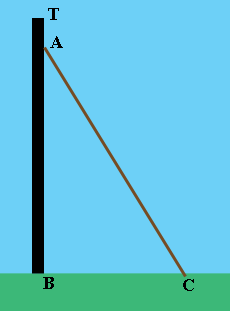SEARCH HOMEMath Central Quandaries & QueriesQuestion from cynthia, a student: 20 foot rope is tied 2 feet from the top of a pole and secured 8 feet from the base of the pole....How tall is the pole?Hi Cynthia,

Did you draw a diagram?$AC$ is 20 feet, $BC$ is 8 feet and $TA$is 2 feet. Suppose the height of the pole, $TB$ is $h$ feet. What is the distance from $A$ to $B?$ Triangle $ABC$ is a right triangle. What does Pythagoras tell you?

PennyMath Central is supported by the University of Regina and The Pacific Institute for the Mathematical Sciences.